Home Practice
For learners and parents For teachers and schools
Textbooks
Full catalogue
Pricing SupportLog in

We think you are located in United States. Is this correct?

# Area and perimeter of triangles

## 16.5 Area and perimeter of triangles

The perimeter of a triangle is the sum of the lengths of its three sides.

For a scalene triangle with three sides of different lengths:

$\text{Perimeter } = a + b + c$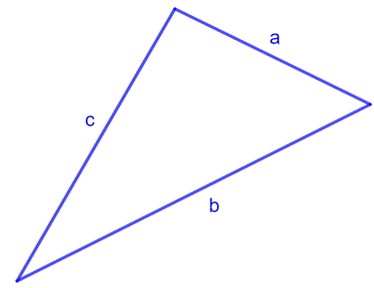For an isosceles triangle with two sides of equal length:

$\text{Perimeter } = 2a + b$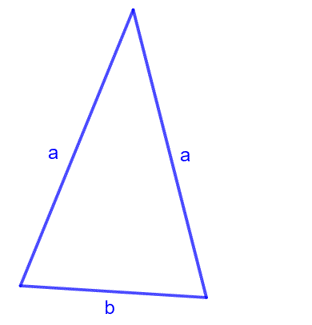For an equilateral triangle with all three sides equal in length:

$\text{Perimeter } = 3a$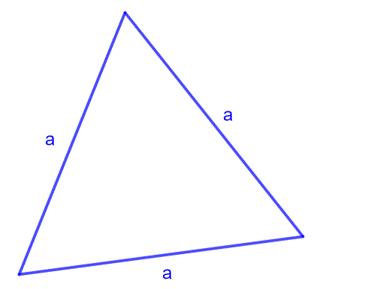## Worked example 16.10: Calculating the perimeter of a triangle

Find the perimeter of the triangle.### Calculate the perimeter of the triangle.

The triangle is a scalene triangle with three sides of different lengths.

$\text{Perimeter } = 7 + 8 + 9 = 24 \text{ cm}$

$$\text{Perimeter of the triangle} = 24 \text{ cm}$$.

The formula for the area of a triangle is half the length of the base ($$b$$) times the perpendicular height ($$h$$) of the triangle.

Area of a triangle $$= \frac{1}{2} (b \times h)$$

We can choose any side of a triangle to be the base of the triangle. The perpendicular height of a triangle is the perpendicular line drawn from the opposite vertex to the base of the triangle. If a triangle is an obtuse-angled triangle, we can extend the base of the triangle so that the perpendicular height can be drawn.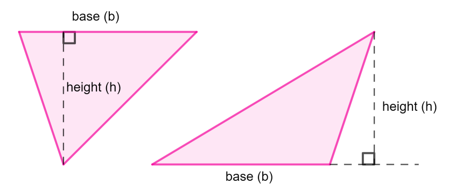## Worked example 16.11: Calculating the area of a triangle by extending the base

Calculate the area of $$\triangle DEF$$.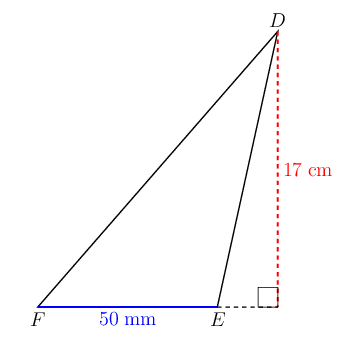### Convert the lengths to the same unit.

The diagram shows that $$FE = 50 \text{ mm}$$ and $$DE = 17 \text{ cm}$$. Before we can calculate the area of the triangle, we need to convert the lengths to the same unit.

$$10 \text{ mm} = 1 \text{ cm}$$, so we can divide $$FE$$ by $$10$$ to get a length in centimetres:

$FE = 50 \text{ mm} = \frac{50}{10} = 5 \text{ cm}$

### Calculate the area of the triangle.

\begin{align} \text{Area of a triangle } &= \frac{1}{2} (b \times h) \\ \text{Area } \triangle DEF &= \frac{1}{2} (5 \times 17) \\ &= \text{42,5} \end{align}

$$\text{Area } \triangle DEF = \text{42,5} \text{ cm}^2$$.

## Worked example 16.12: Calculating the area of a triangle using the correct perpendicular height

In $$\triangle XYZ$$, $$YZ = \text{7} \text{ cm}, YT = \text{6,5} \text{ cm}$$ and $$XS = \text{6} \text{ cm}$$. Determine the area of $$\triangle XYZ$$.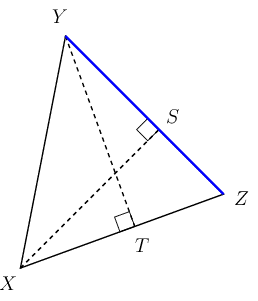### Identify the perpendicular height of the triangle for base $$YZ$$.

We know the length of the side $$YZ$$ so we can take $$YZ$$ to be the base of the triangle. Vertex $$X$$ lies opposite the base $$YZ$$, so the correct perpendicular height for base $$YZ$$ is $$XS$$.

Note that $$YT$$ is the perpendicular height of the triangle for base $$XZ$$.

### Calculate the area of the triangle.

\begin{align} \text{Area of a triangle } &= \frac{1}{2} (b \times h) \\ \text{Area } \triangle XYZ &= \frac{1}{2} (7 \times 6) \\ &= 21 \end{align}

$$\text{Area } \triangle XYZ = 21 \text{ cm}^2$$.